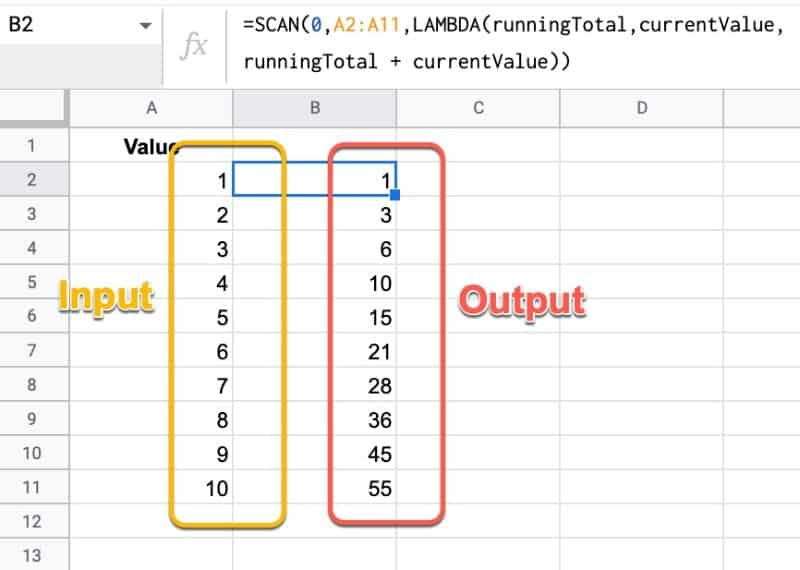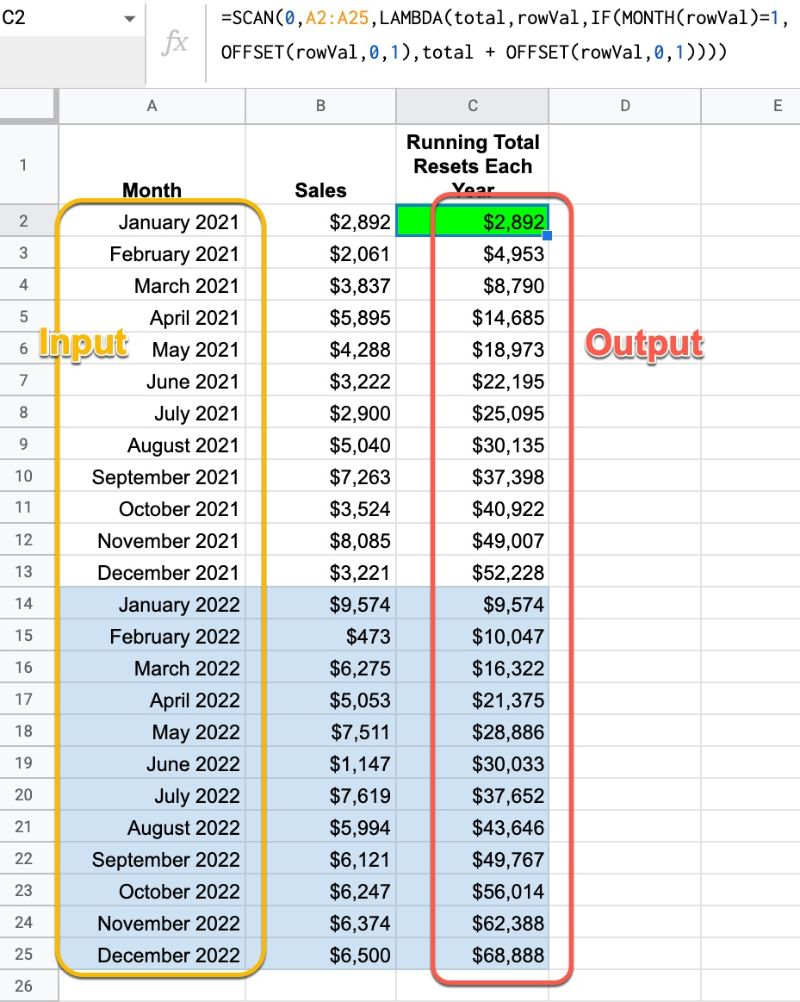# SCAN Function in Google Sheets – LAMBDA Helper Function

The SCAN function in Google Sheets scans an array and applies a LAMBDA function to each value, moving row by row. The output is an array of intermediate values obtained at each step.

It’s hard to understand without seeing an example, so let’s do that.

The most straightforward SCAN formula is to create a running total calculation on a column of numbers.

The formula is:

`=SCAN(0,A2:A11,LAMBDA(runningTotal,currentValue,runningTotal + currentValue))`

Which produces this array output:The lambda simply adds together the current running total and the new value, then returns the answer to the output array.

When all of the values from the input array have been considered, the output array is returned.

🔗 Get this example and others in the template at the bottom of this article.

## SCAN Function Syntax

`=SCAN(initial_value, array_or_range, lambda)`

It takes 3 arguments:

`initial_value`

This is the starting value of the accumulator.

`array_or_range`

This is the input array or range of data to be reduced.

`lambda`

The custom LAMBDA function that will be applied to each value in the input range. The LAMBDA function must have 2 name arguments and 1 function expression.

The first name will evaluate to the current result in the accumulator. The second name resolves to the current value of the input array.

### SCAN Function Notes

• The output array is the same size as the input array.
• The SCAN function is a spill formula, i.e. it requires enough space for the array output to fully expand.

## SCAN Function Example: Annual Running Total

Suppose we have monthly revenue data for our business for a number of years and we want to calculate a running total for each year. We want the running total to reset for each year.

We can do that with this SCAN formula:

`=SCAN(0,A2:A25,LAMBDA(total,rowVal,IF(MONTH(rowVal)=1,OFFSET(rowVal,0,1),total + OFFSET(rowVal,0,1))))`

The output of this formula is shown in column C:Let’s break down this formula, using the onion framework approach.

rowVal represents the current value from the input array, i.e. a date.

So the IF function statement is testing whether the month is January or not:

`IF(MONTH(rowVal)=1`

If it is a January month, we want to reset the running total, and start a new one with the January value, which we do as follows:

`IF(MONTH(rowVal)=1,OFFSET(rowVal,0,1)`

The OFFSET(rowVal,0,1) just grabs the value from column B on the same row, i.e. the revenue value.

Now, if the month is not January, then we want to add the new month value to the existing running total, which we do in the FALSE part of the IF statement:

`IF(MONTH(rowVal)=1,OFFSET(rowVal,0,1),total + OFFSET(rowVal,0,1))`

This is the formula expression for our LAMBDA:

`LAMBDA(total,rowVal,IF(MONTH(rowVal)=1,OFFSET(rowVal,0,1),total + OFFSET(rowVal,0,1)))`

Finally, we can plug this into the SCAN function. We set the initial value to 0 and select the date column as our input array:

`=SCAN(0,A2:A25,LAMBDA(total,rowVal,IF(MONTH(rowVal)=1,OFFSET(rowVal,0,1),total + OFFSET(rowVal,0,1))))`

## SCAN Function Template

Feel free to make a copy: File > Make a copy…

If you can’t access the template, it might be because of your organization’s Google Workspace settings.

In this case, right-click the link to open it in an Incognito window to view it.

See the LAMBDA function and other helper functions MAP, REDUCE, MAKEARRAY, etc. here:

Named Functions and New Functions In Google Sheets For 2022

## 3 thoughts on “SCAN Function in Google Sheets – LAMBDA Helper Function”

1.MikeJ says:

Named functions and Lambda were activated in my Workspace account yesterday. None of the Lambda helper functions have been activated, nor has Xlookup. Nothing so far in my regular Google account.

2.Daniel says:

Might be nice to tie the fact that SCAN is REDUCE, with the result of each step of the REDUCE included in the output array.

3.reg says:

Is there a way to scan from the bottom of the range up?

I’m working a lot with running totals, but we like to have the latest time periods at the top in descending order.